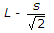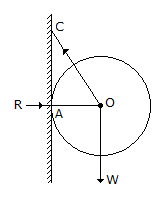# Civil Engineering - UPSC Civil Service Exam Questions

26.

The effective length of a fillet weld of length 'L' and size 's' is given by

 A. L - s B. L - 2s C.D. L

Explanation:

No answer description available for this question. Let us discuss.

27.

Two reservoirs are connected by two pipes A and B of same and length in series. If the diamerer of A is 30% larger than that of B, what is the ratio of head loss in A to that of B ?

 A. 0.77 B. 0.59 C. 0.5 D. 0.27

Explanation:

No answer description available for this question. Let us discuss.

28.

A smooth sphere of weight W is supported in contact with a smooth vertical wall by a string fastened to a point on its surface, the other end being attached to a point in the wall as shown in the given figure. If the length of the string is equal to the diameter of the sphere, then the tension in the string and the reaction of the wall respectively will beA. 4W/3, 2W/3 B. 2W/3, W/3 C. 5W/3, W/3 D. 3W/3, W/3

Explanation:

No answer description available for this question. Let us discuss.

29.

In an external focussing tacheometer, the fixed interval between the stadia hairs is 5 mm; the focal length of the objective is 25 cm ; and the distance of the vertical axis of the instrument from the optical centre of the objective is 15 cm. The constants of the tacheometer are

 A. 50 ; 0.40 m B. 50 ; 0.25 m C. 100 ; 0.40 m D. 30 ; 0.10 m

Explanation:

No answer description available for this question. Let us discuss.

30.

On a single rail track, goods trains loaded with heavy iron material run starting from A to B and then empty wagons run from B to A.
The amount of creep in the rails

 A. will be more in the direction of B to A B. will be more in the direction of A to B C. will be maximum in the direction of A and B D. cannot be determined from the given data